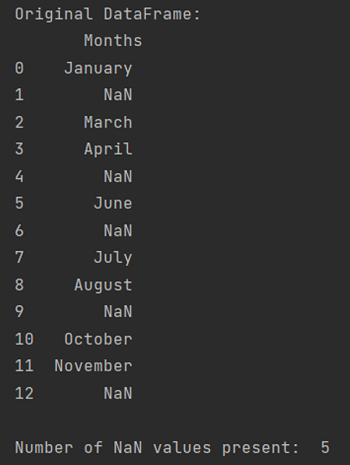# Count Column-wise NaN Values in Pandas DataFrame

In this tutorial, we will learn how can we count the column-wise NaN values in a Pandas DataFrame? By Pranit Sharma Last updated : April 19, 2023

## What are NaN Values?

While creating a DataFrame or importing a CSV file, there could be some NaN values in the cells. NaN values mean "Not a Number" which generally means that there are some missing values in the cell. To deal with this type of data, you can either remove the particular row (if the number of missing values is low) or you can handle these values. For handling these values, you might need to count the number of NaN values.

## How to Count Column-wise NaN Values?

To count the column-wise NaN values, simply use the df["column"].isnull().sum(), it will check for the NaN value in the given column and returns the sum (count) of all the NaN values in the given column of Pandas DataFrame.

### Syntax

The syntax for counting column-wise value in DataFrame:

```DataFrame["Column_Name"].isnull().sum()
```

Let us understand with the help of an example.

## Python Program to Count Column-wise NaN Values in Pandas DataFrame

```# Importing Pandas package
import pandas as pd

# Importing Numpy package
import numpy as np

# Creating a dictionary
dict = {
"Months": [
"January",
np.NaN,
"March",
"April",
np.nan,
"June",
np.nan,
"July",
"August",
np.nan,
"October",
"November",
np.NaN,
]
}

# Creating the dataframe
df = pd.DataFrame(dict)

# Viewng the original DataFrame
print("Original DataFrame:\n", df, "\n")

# Applying the isnull method
nan = df["Months"].isnull().sum()

# printing the number of NaN values present
print("Number of NaN values present: ", nan)
```

### Output

```Original DataFrame:
Months
0    January
1        NaN
2      March
3      April
4        NaN
5       June
6        NaN
7       July
8     August
9        NaN
10   October
11  November
12       NaN

Number of NaN values present:  5
```

### Output Screenshot